Open In App
Related Articles
• NCERT Solutions for Class 9 Maths

# Class 9 NCERT Solutions – Chapter 9 Areas of Parallelograms And Triangles – Exercise 9.2

### Question 1: In given Fig. ABCD is a parallelogram, AE ⊥ DC and CF ⊥ AD. If AB = 16 cm, AE = 8 cm and CF = 10 cm, find AD?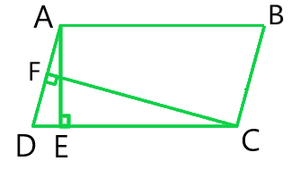Solution:

As Given in the Question,

AB = CD = 16 cm (Opposite sides of a parallelogram)

CF = 10 cm and AE = 8 cm

Now, As we have studied in this Chapter we know,

Area of Parallelogram = Base x Altitude

⇒ CD × AE = AD × CF

⇒ 16 × 8 = AD × 10

### Question 2: If E, F, G, and H are respectively the mid-points of the sides of a parallelogram ABCD, show that ar (EFGH) = 1/2 ar(ABCD)?

Solution: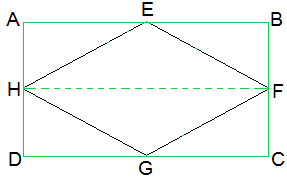As Given in the Question ,

E, F, G and H are the mid-points of the sides of a parallelogram ABCD respectively.

To Prove,

ar (EFGH) = ½ ar(ABCD)

First, we have to do some construction

join H to E

Proof:

As we know,

AD || BC and AD = BC (Opposite sides of a parallelogram)

⇒ ½ AD = ½ BC

As H and F are the mid points of AD and BC

AH || BF and and DH || CF

Therefore,

AH = BF and DH = CF (H and F are mid points)

∴ ABFH and HFCD are parallelograms.

As we know that, ΔEFH and parallelogram ABFH, both lie on the same base FH and ΔEFH lie in-between the same parallel lines AB and HF.

Therefore,

Area of EFH = ½ Area of ABFH — (i)

And, Area of GHF = ½ Area of HFCD — (ii)

Now, Adding (i) and (ii) we get,

Area of ΔEFH + Area of ΔGHF = ½ Area of ABFH + ½ Area of HFCD

⇒ Area of EFGH = Area of ABFH

∴ ar (EFGH) = ½ ar(ABCD)

### Question 3: P and Q are any two points lying on the sides DC and AD respectively of a parallelogram   ABCD. Show that ar(APB) = ar(BQC)?

Solution: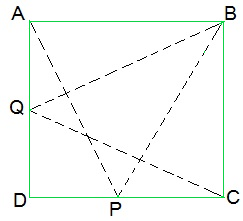ΔAPB and parallelogram ABCD lie on the same base AB and ΔAPB lie in-between same parallel AB and DC.

Now, As we know that

ar(ΔAPB) = ½ ar(parallelogram ABCD) — (i)

Similarly,

ar(ΔBQC) = ½ ar(parallelogram ABCD) — (ii)

From (i) and (ii), we have

ar(ΔAPB) = ar(ΔBQC)

Hence proved,

### Question 4: In the Figure, P is a point in the interior of a parallelogram ABCD. Show that

(i) ar(APB) + ar(PCD) = ½ ar(ABCD)

(ii) ar(APD) + ar(PBC) = ar(APB) + ar(PCD)

[Hint : Through P, draw a line parallel to AB.]Solution:

Given: P is a point in the interior of the parallelogram ABCD

To prove: Area(APB) + Area(PCD) = ½ Area(ABCD)

Construction:

Through P, draw a line EF parallel to AB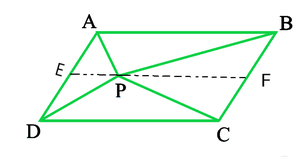Proof:

(i) In a parallelogram,

AB || EF (by construction) — (i)

∴AD || BC ⇒ AE || BF — (ii)

From equations (i) and (ii),

ABFE is a parallelogram.

Now,

ΔAPB and parallelogram ABFE are lying on the same base AB and ΔAPB lie in-between the same parallel lines AB and EF.

∴ ar(ΔAPB) = ½ ar(ABFE) — (iii)

also,

ΔPCD and parallelogram CDEF are lying on the same base CD and ΔPCD lie in-between the same parallel lines CD and EF.

∴ ar(ΔPCD) = ½ ar(CDEF) — (iv)

Adding equations (iii) and (iv) we get,

ar(ΔAPB) + ar(ΔPCD) = ½ [ar(ABFE)+ar(CDEF)]

⇒ ar(APB)+ ar(PCD) = ½ ar(ABCD)

Hence Proved

(ii) Construction:

Through P, draw a line GH parallel to AB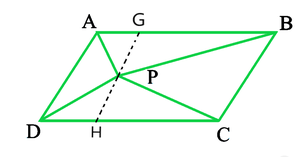In the parallelogram,

AD || GH (by construction) — (i)

∴AB || CD ⇒ AG || DH — (ii)

From equations (i) and (ii) we get,

AGDH is a parallelogram.

Now,

ΔAPD and parallelogram AGHD are lying on the same base AD and ΔAPD lie in-between the same parallel lines AD and GH.

∴ar(ΔAPD) = ½ ar(AGHD) — (iii)

also,

ΔPBC and parallelogram BCHG are lying on the same base BC and ΔPBC lie in-between the same parallel lines BC and GH.

∴ar(ΔPBC) = ½ ar(BCHG) — (iv)

Adding equations (iii) and (iv) we get,

ar(ΔAPD) + ar(ΔPBC) = ½ {ar(AGHD) + ar(BCHG)}

⇒ ar(APD) + ar(PBC) = ar(APB) + ar(PCD)

Hence Proved

### Question 5: In the Figure, PQRS and ABRS are parallelograms and X is any point on side BR. Show that:

(i) ar (PQRS) = ar (ABRS)

(ii) ar (AXS) = ½ ar (PQRS)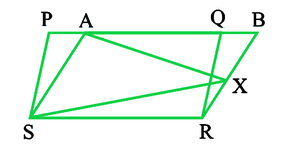Solution:

Given:  PQRS and ABRS are parallelograms and X is any point on side BR

To Prove: (i) ar (PQRS) = ar (ABRS)

(ii) ar (AXS) = ½ ar (PQRS)

Proof:

(i) In ΔPSA and ΔQRB,

∠ SPA = ∠ RQB  — (i) (Corresponding Angles from PS || QR and traversal PB

∠ PAS = ∠ QBR — (ii) (Corresponding Angles from AS || BR and traversal PB

∠ PSA = ∠ QRB — (iii) (Angle Sum Property of triangle)

Also, PS = QR  — (iv) (Opposite sides of Parallelogram PQRS)

In view of (i), (iii) and (iv),

ΔPSA ≅  ΔQRB — (v) (By ASA Rule)

∴ Area(ΔPSA) = Area(ΔQRB)  — (vi)

∴ Congruent figures have Equal Area

Now, ar(PQRS) = Area(ΔPSA) + Area(AQRS)

= Area(ΔQRB) + Area(AQRS)   —–|| Using (vi)

= ar(ABRS)

∴ar (PQRS) = ar (ABRS)

Hence Proved

(ii) ΔAXS and Parallelogram ABRS are on the Same base As and between the Same parallels AS and BR

∴ Area(ΔAXS) = ½ Area(Parallelogram ABRS)

= ½ {Area(AQRS) + Area(ΔQRB)}

=  ½ Area(Parallelogram PQRS)

Hence Proved

### Question 6: A farmer was having a field in the form of a parallelogram PQRS. She took any point A on RS and joined it to points P and Q. In how many parts the fields are divided? What are the shapes of these parts? The farmer wants to sow wheat and pulses in equal portions of the field separately. How should she do it?

Solution: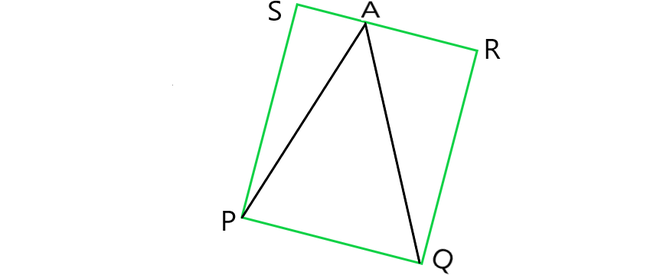The field is divided into three parts each in triangular shape.

Let, ΔPSA, ΔPAQ and ΔQAR be the triangles.

Area of (ΔPSA + ΔPAQ + ΔQAR) = Area of PQRS — (i)

Area of ΔPAQ = ½ area of PQRS — (ii)

Here, the triangle and parallelogram are on the same base and in-between the same parallel lines.

From (i) and (ii) we get,

Area of ΔPSA + Area of ΔQAR = ½ area of PQRS — (iii)

From (ii) and (iii), we can conclude that,

The farmer must sow wheat or pulses in ΔPAQ or either in both ΔPSA and ΔQAR.